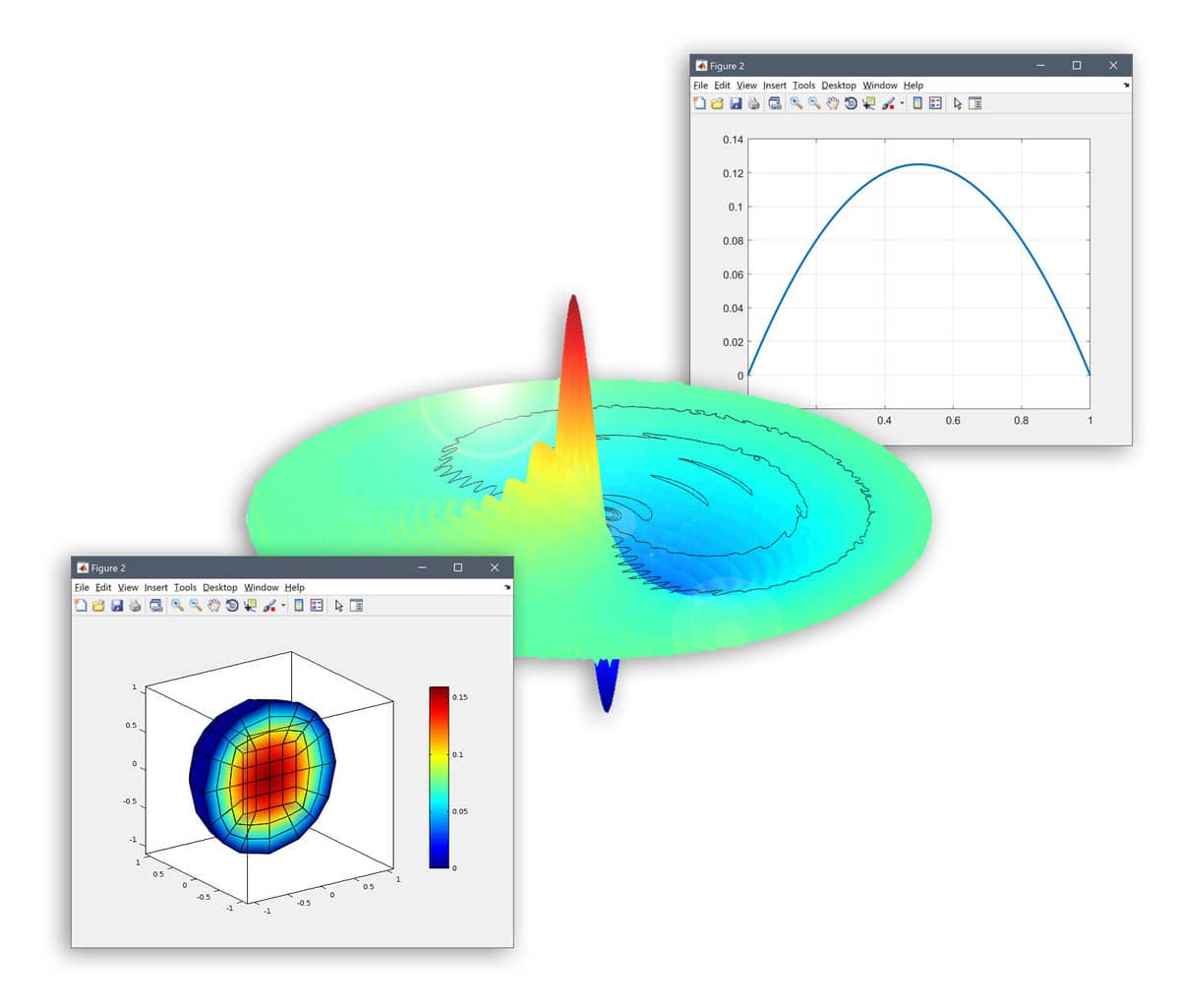# Multiphysics Modeling and Simulation## Multiphysics

Multiphysics is commonly referred to as the process of computer simulation of multiple coupled and interacting physical phenomena. Multiphysics simulations are becoming increasingly used in computer aided engineering (CAE), virtual prototyping, and product design fields. This could for example be heat generation due to friction leading to stresses, fluid flow transporting reacting chemical species, or temperature variations due to electrical fields.

FEATool multiphysics solves coupled continuum mechanics and partial differential equations (PDE) using a fully coupled monolithic method. In contrast to typical segregated and splitting approaches found in other solvers, this approach leads to better non-linear convergence properties and an overall more robust and stable solution approach.

## Heat and Mass Transfer

Heat transport by thermal conduction in solids and/or convection in fluids is modeled with the heat transfer equation. For multiphysics applications, the temperature field can be coupled to other physics such as structural mechanics applications for thermal stresses, or fluid flow to account for buoyancy effects.

Mass transfer is treated by the analogous convection, diffusion, and reaction equation to account for mass diffusion and chemical reactions. Full two way multiphysics couplings are also sometimes present accounting for the convective transport of concentration fields by the fluid flow.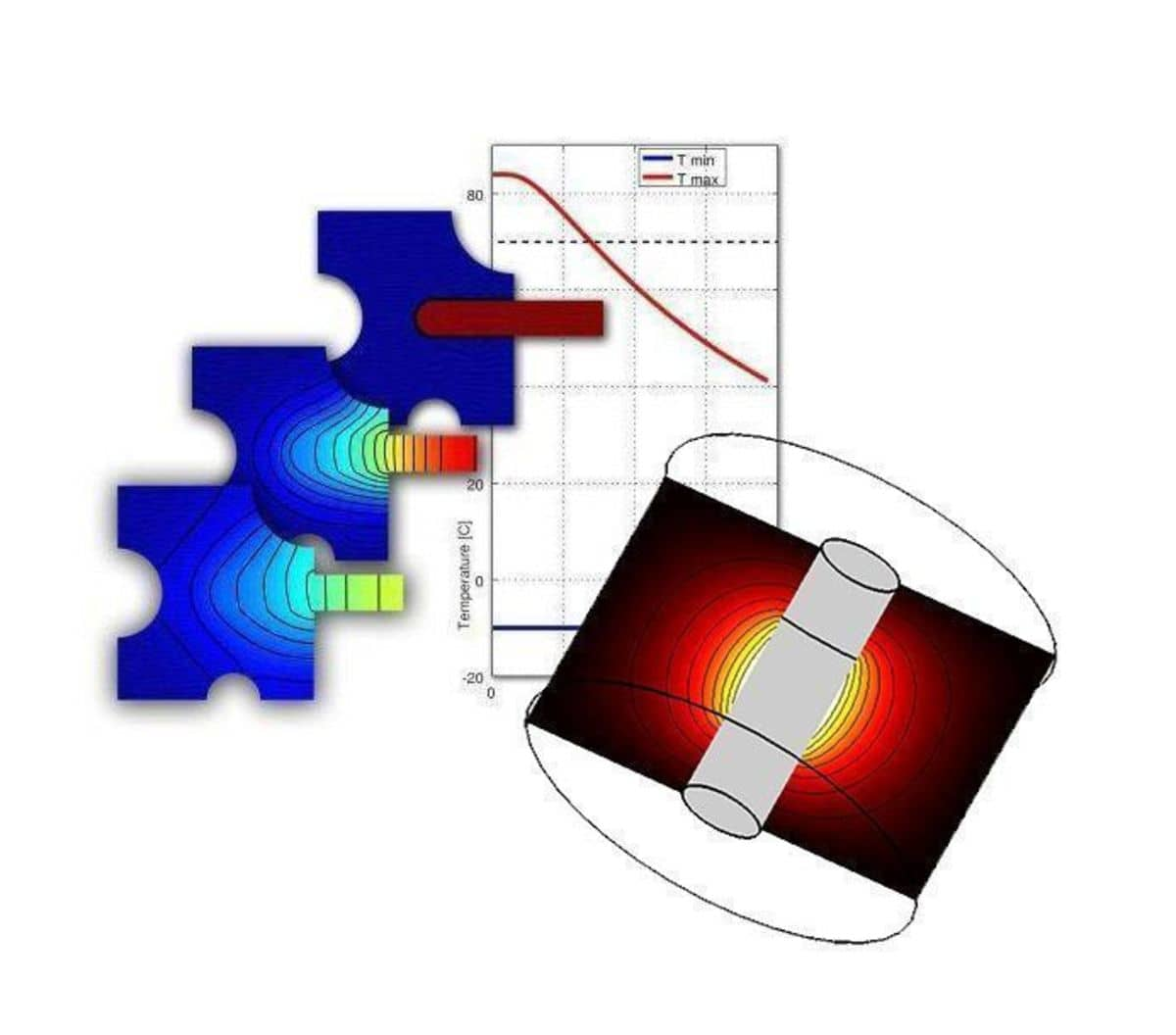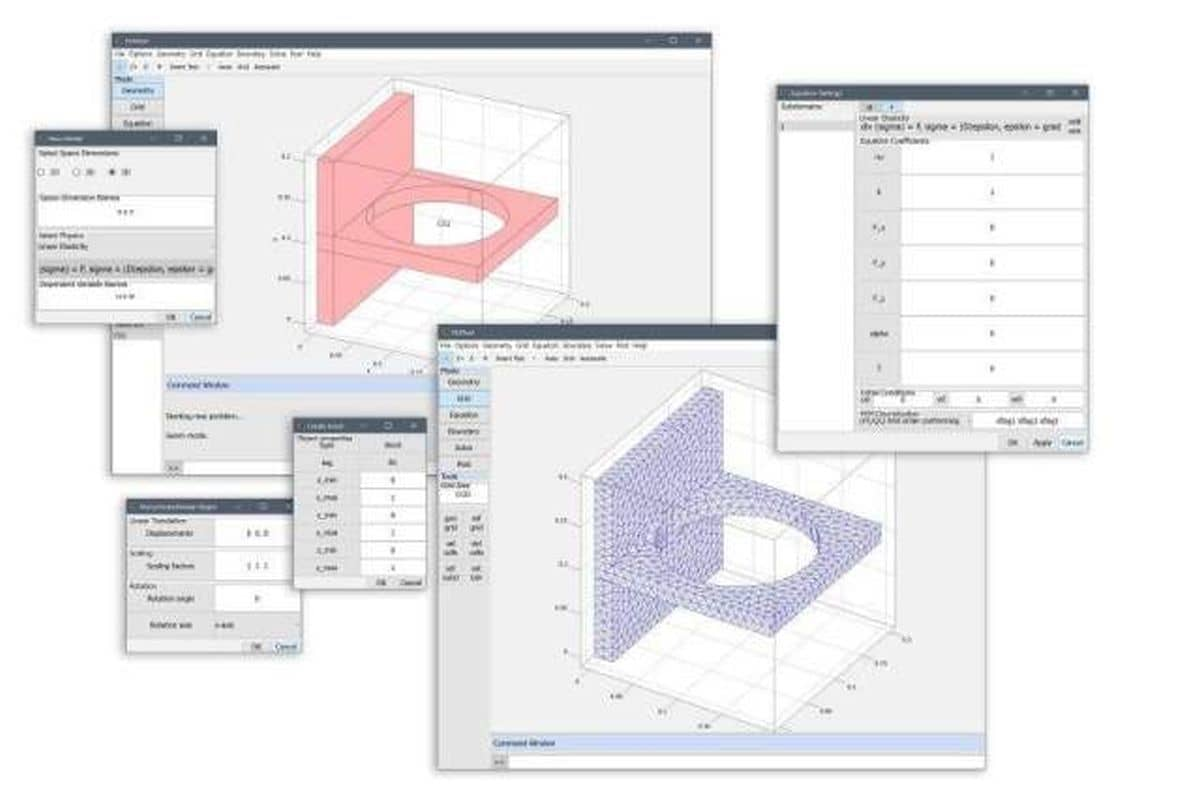## Structural Mechanics

Structural mechanics and mechanical engineering simulations are used to determine stresses, strains, displacements, and failure modes for objects and structures under varying load conditions.

Multiphysics simulations for stress-strain relations often also involve heating effects giving rise to temperature induced stresses, such as during braking procedures. Also fluid-structure interaction (FSI) whereby a fluid exerts significant forces on solid objects can often be found in pharmaceutical and bio-mechanical applications.

## Fluid Mechanics

Computational fluid dynamics (CFD) simulations are typically used to model the behavior of fluids in motion. CFD application areas span everything from large scale aerospace, aerodynamic, geo and ocean engineering, to automotive, manufacturing and processing industry, and small scale bio and micro-fluidic applications.

In multiphysics applications the effects of fluid flow fields can often be very significant. For example, heat can be transported away by the fluid field such as in heat exchangers, chemical reactions might occur at significantly different rates in fluid stagnation zones, and fluids exert significant forces on and interact with surrounding objects such as in fluid-structure interaction applications.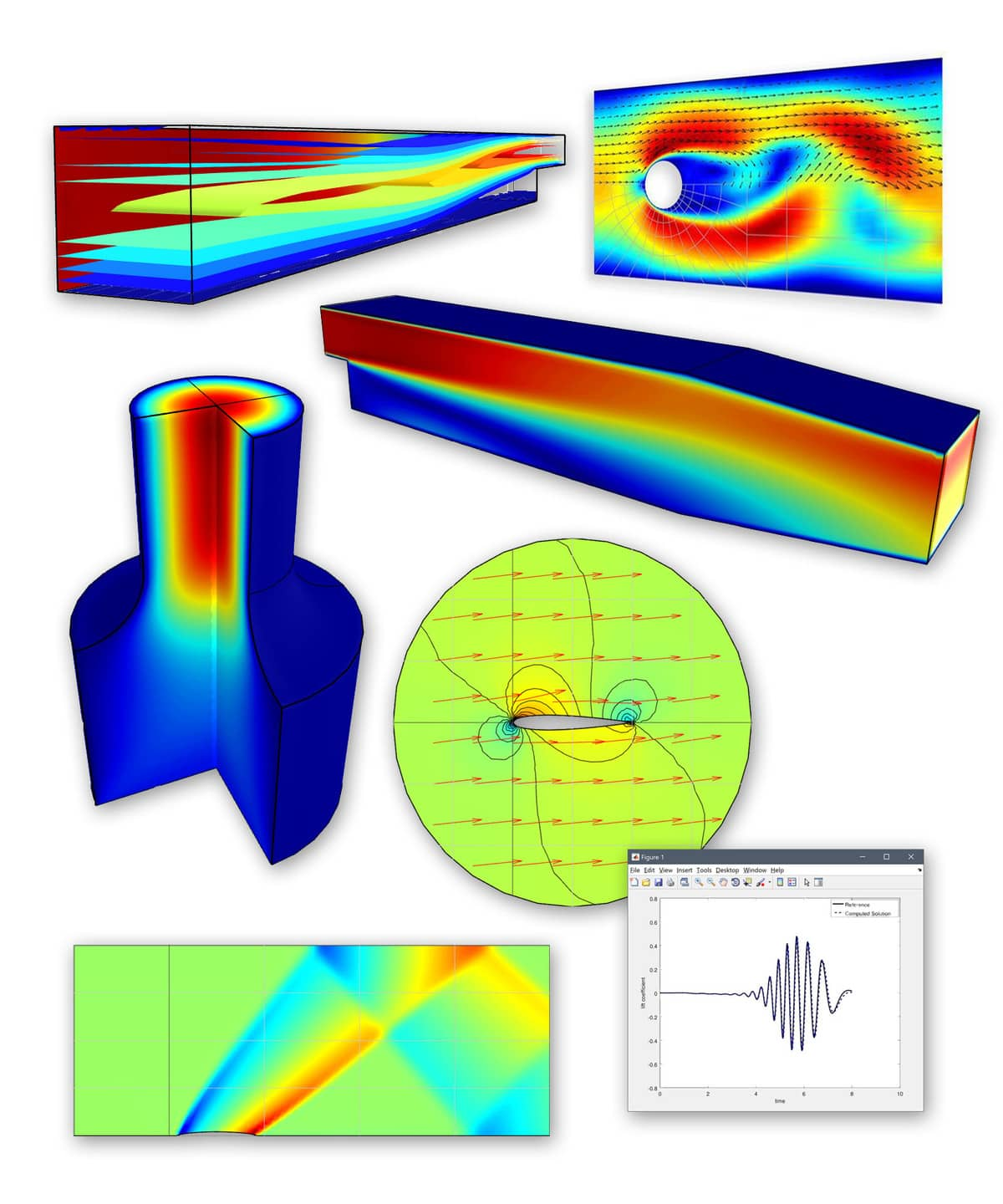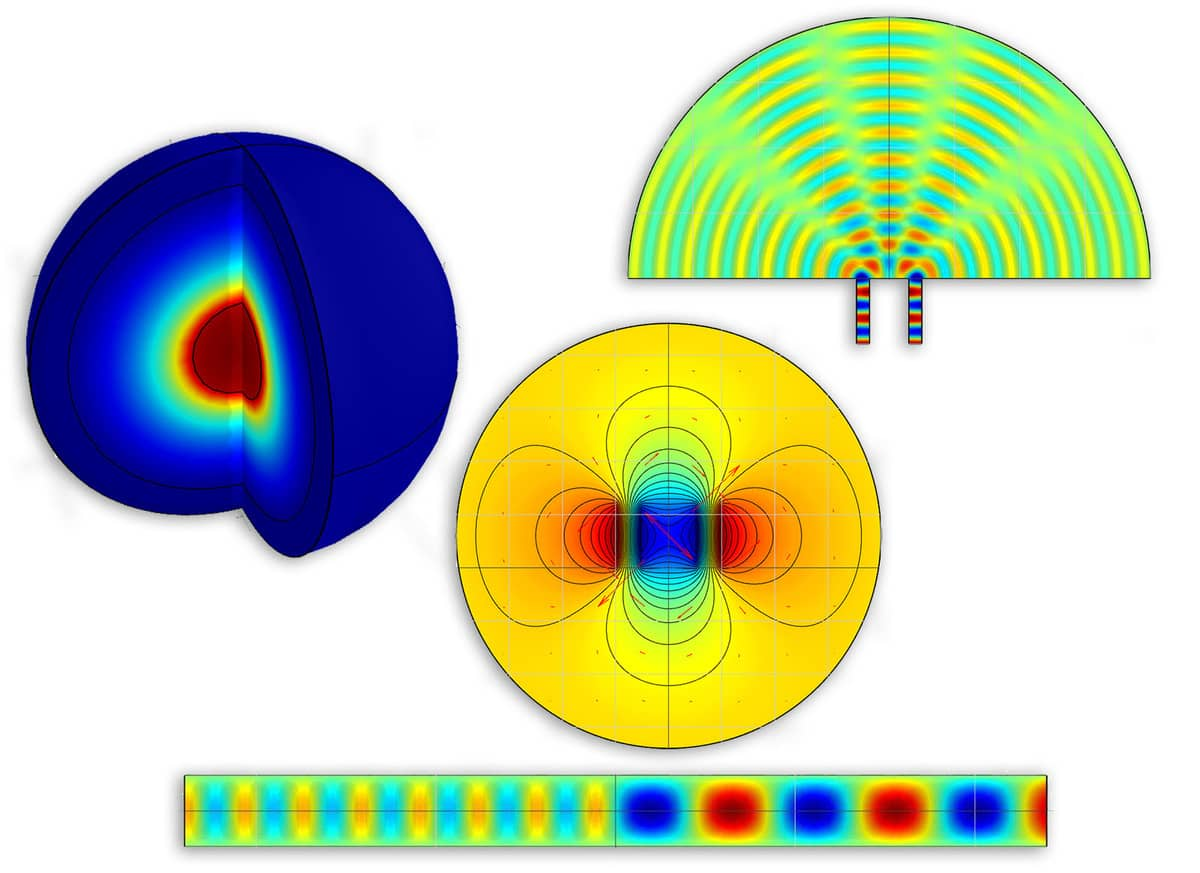## Electromagnetics

Electromagnetic forces and field effects as for example found in antennas, wave guides, magnets, and electrical components are typically modeled with different forms of Maxwell's equations.

In multiphysics applications electromagnetic fields can generate forces on conductive objects, and also give rise to resistive and inductive heating effects.

## Partial Differential Equations

Partial differential equations (PDE) are typically the building blocks in continuum mechanics and multiphysics modeling applications. The governing equations for the application areas above can often be reduced to the a form of classic and prototypical PDEs such as the Poisson's, Laplace, wave, and convection and diffusion equations.

The pre-defined physics modes in FEATool Multiphysics hides the work of defining PDEs and multiphysics couplings. These governing equation systems can then be coupled and automatically and efficiently solved together.